JEE  >  Test: Comprehension Based Questions: Definite Integrals and Applications of Integrals

# Test: Comprehension Based Questions: Definite Integrals and Applications of Integrals

Test Description

## 16 Questions MCQ Test Maths 35 Years JEE Main & Advanced Past year Papers | Test: Comprehension Based Questions: Definite Integrals and Applications of Integrals

Test: Comprehension Based Questions: Definite Integrals and Applications of Integrals for JEE 2023 is part of Maths 35 Years JEE Main & Advanced Past year Papers preparation. The Test: Comprehension Based Questions: Definite Integrals and Applications of Integrals questions and answers have been prepared according to the JEE exam syllabus.The Test: Comprehension Based Questions: Definite Integrals and Applications of Integrals MCQs are made for JEE 2023 Exam. Find important definitions, questions, notes, meanings, examples, exercises, MCQs and online tests for Test: Comprehension Based Questions: Definite Integrals and Applications of Integrals below.
Solutions of Test: Comprehension Based Questions: Definite Integrals and Applications of Integrals questions in English are available as part of our Maths 35 Years JEE Main & Advanced Past year Papers for JEE & Test: Comprehension Based Questions: Definite Integrals and Applications of Integrals solutions in Hindi for Maths 35 Years JEE Main & Advanced Past year Papers course. Download more important topics, notes, lectures and mock test series for JEE Exam by signing up for free. Attempt Test: Comprehension Based Questions: Definite Integrals and Applications of Integrals | 16 questions in 30 minutes | Mock test for JEE preparation | Free important questions MCQ to study Maths 35 Years JEE Main & Advanced Past year Papers for JEE Exam | Download free PDF with solutions
 1 Crore+ students have signed up on EduRev. Have you?
Test: Comprehension Based Questions: Definite Integrals and Applications of Integrals - Question 1

### Let the definite integral be defined by the formula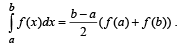For more accurate result for c ∈ (a, b), we can use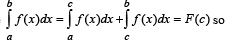that for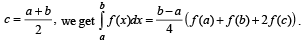Q.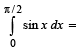Detailed Solution for Test: Comprehension Based Questions: Definite Integrals and Applications of Integrals - Question 1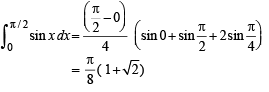Test: Comprehension Based Questions: Definite Integrals and Applications of Integrals - Question 2

### Let the definite integral be defined by the formulaFor more accurate result for c ∈ (a, b), we can usethat forQ.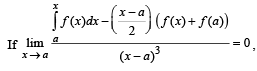then f(x) is of maximum degree

Detailed Solution for Test: Comprehension Based Questions: Definite Integrals and Applications of Integrals - Question 2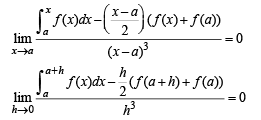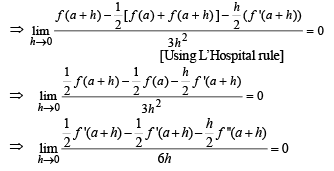[Using L’Hospital rule]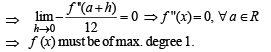Test: Comprehension Based Questions: Definite Integrals and Applications of Integrals - Question 3

### Let the definite integral be defined by the formulaFor more accurate result for c ∈ (a, b), we can usethat forQ.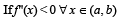and c is a point such that a < c < b, and (c, f(c)) is the point lying on the curve for which F(c) is maximum, then f '(c) is equal to

Detailed Solution for Test: Comprehension Based Questions: Definite Integrals and Applications of Integrals - Question 3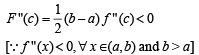∴ F (c) is max. at the point (c, f (c)) where F’ (c) = 0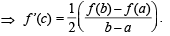(For 4-6). Given the implicit function y3 – 3y + x = 0 For x ∈ (–∞, –2) ∪ (2,∞) it is y = f (x) real valued differentiable function, and for x ∈ (–2, 2) it is y = g(x) real valued differentiable function.

Test: Comprehension Based Questions: Definite Integrals and Applications of Integrals - Question 4

PASSAGE - 2

Consider the functions defined implicitly by the equation y3 – 3y + x = 0 on various intervals in the real line. If x ∈(-∞, - 2) ∪ (2,∞) , the equation implicitly defines a unique real valued differentiable function y = f (x). If x ∈(-2, 2) , the equation implicitly defines a unique real valued differentiable function y = g(x) satisfying g(0) = 0.

Q.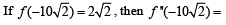Detailed Solution for Test: Comprehension Based Questions: Definite Integrals and Applications of Integrals - Question 4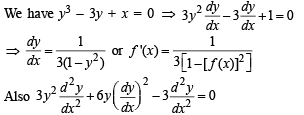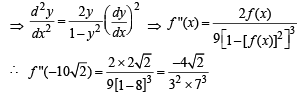Test: Comprehension Based Questions: Definite Integrals and Applications of Integrals - Question 5

PASSAGE - 2

Consider the functions defined implicitly by the equation y3 – 3y + x = 0 on various intervals in the real line. If x ∈(-∞, - 2) ∪ (2,∞) , the equation implicitly defines a unique real valued differentiable function y = f (x). If x ∈(-2, 2) , the equation implicitly defines a unique real valued differentiable function y = g(x) satisfying g(0) = 0.

Q. The area of the region bounded by the curve y = f (x), the x-axis, and the lines x = a and x = b, where -∞ < a <b <-2 , is

Detailed Solution for Test: Comprehension Based Questions: Definite Integrals and Applications of Integrals - Question 5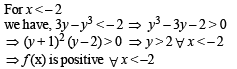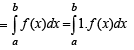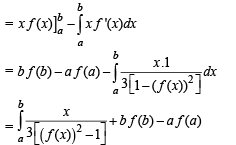Test: Comprehension Based Questions: Definite Integrals and Applications of Integrals - Question 6

PASSAGE - 2

Consider the functions defined implicitly by the equation y3 – 3y + x = 0 on various intervals in the real line. If x ∈(-∞, - 2) ∪ (2,∞) , the equation implicitly defines a unique real valued differentiable function y = f (x). If x ∈(-2, 2) , the equation implicitly defines a unique real valued differentiable function y = g(x) satisfying g(0) = 0.

Q.Detailed Solution for Test: Comprehension Based Questions: Definite Integrals and Applications of Integrals - Question 6

For y = g(x), we have y3 – 3y + x = 0
⇒ [g (x) ]3 - 3[ g (x)] + x=0 ...(1)
Putting x = –x, we get
⇒ [g (-x)]3 - 3[ g (-x)] - x = 0 ...(2)
Adding equations (1) and (2) we get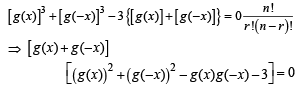For g(0) = 0, we should have g(x) + g(–x) = 0
[∵ From other factor we get g(0) = ±√3]

⇒ g(x) is an odd function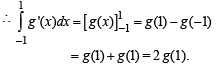Test: Comprehension Based Questions: Definite Integrals and Applications of Integrals - Question 7

PASSAGE - 3

Consider the function f : ( -∞,∞) →(-∞,∞) defined by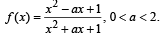Q. Which of the following is true?

Detailed Solution for Test: Comprehension Based Questions: Definite Integrals and Applications of Integrals - Question 7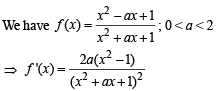⇒ (x2 + ax + 1) 2 f '(x) = 2a(x2- 1)
⇒ (x2 + ax +1)2 f "(x) + 2(x2 + ax+1)
(2 x + a) f '(x)=4ax ...(1)
Putting x = –1 in equation (1), we get
(2 - a2) f ''(-1) =-4a …(2)
Putting x = 1 in equation (1), we get
(2 + a)2 f "(1)=4a ...(3)
Adding equations (2) and (3), we get
(2 + a)2 f "(1) + (2 - a)2f "(-1)= 0

Test: Comprehension Based Questions: Definite Integrals and Applications of Integrals - Question 8

PASSAGE - 3

Consider the function f : ( -∞,∞) →(-∞,∞) defined byQ. Which of the following is true?

Detailed Solution for Test: Comprehension Based Questions: Definite Integrals and Applications of Integrals - Question 8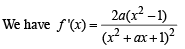f '(x) = 0 ⇒ x = –1, 1 are the critical points.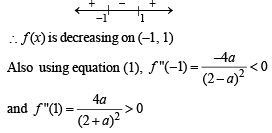∴ x = – 1 is a point of local maximum
and x = 1 is a point of local minimum.

Test: Comprehension Based Questions: Definite Integrals and Applications of Integrals - Question 9

PASSAGE - 3

Consider the function f : ( -∞,∞) →(-∞,∞) defined byQ.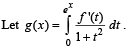Which of the following is true?

Detailed Solution for Test: Comprehension Based Questions: Definite Integrals and Applications of Integrals - Question 9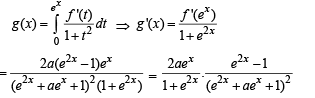Now g '(x) >0 for e2x - 1> 0 ⇒ x > 0
and g '(x) <0 for e2x - 1 < 0 ⇒ x<0
∴ g'(x) is negative on (–∞ , 0) and posit ive on (0, ∞)

Test: Comprehension Based Questions: Definite Integrals and Applications of Integrals - Question 10

PASSAGE - 4

f (x) = 1 + 2x + 3x2 + 4x3.
Let s be the sum of all distinct real roots of f (x) and let t = |s|.

Q. The real numbers lies in the interval

Detailed Solution for Test: Comprehension Based Questions: Definite Integrals and Applications of Integrals - Question 10

f ( x) = 4x3 + 3x2 + 2x+1
∵ f (x) is a cubic polynomial
∴ It has at least one real root.
Also f '(x) =12x2 + 6x+ 2 = 2(6x2 +3x+1)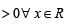∴ f (x) is strictly increasing function
⇒ There is only one real root of f (x) = 0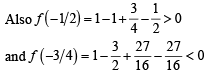∴ Real root lies between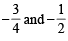and hence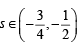Test: Comprehension Based Questions: Definite Integrals and Applications of Integrals - Question 11

PASSAGE - 4

f (x) = 1 + 2x + 3x2 + 4x3.
Let s be the sum of all distinct real roots of f (x) and let t = |s|.

Q. The area bounded by the curve y = f (x) and the lines x = 0, y = 0 and x = t, lies in the interval

Detailed Solution for Test: Comprehension Based Questions: Definite Integrals and Applications of Integrals - Question 11

y = f (x), x = 0,y= 0 and x = t bounds the area as shown in the figure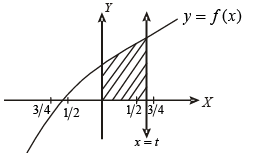∴ Required area is given by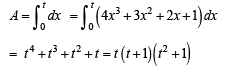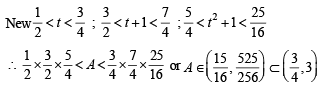Test: Comprehension Based Questions: Definite Integrals and Applications of Integrals - Question 12

PASSAGE - 4

f (x) = 1 + 2x + 3x2 + 4x3.
Let s be the sum of all distinct real roots of f (x) and let t = |s|.

Q. The function f'(x) is

Detailed Solution for Test: Comprehension Based Questions: Definite Integrals and Applications of Integrals - Question 12

f '(x) = 2(6x2 + 3x+ 1)
f ''(x) = 6 4x+ 1) ⇒ Critical point x = – 14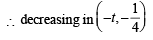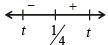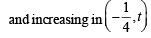Test: Comprehension Based Questions: Definite Integrals and Applications of Integrals - Question 13

PASSAGE - 5

Given that for each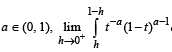dt exists. Let this limit be g(a). In addition, it is given that the function g(a) is differentiable on (0, 1).

Q. The value of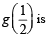Detailed Solution for Test: Comprehension Based Questions: Definite Integrals and Applications of Integrals - Question 13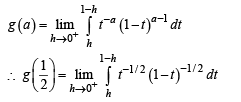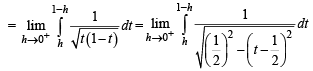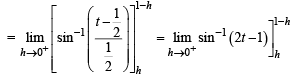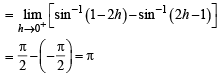Test: Comprehension Based Questions: Definite Integrals and Applications of Integrals - Question 14

PASSAGE - 5

Given that for eachdt exists. Let this limit be g(a). In addition, it is given that the function g(a) is differentiable on (0, 1).

Q.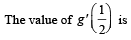Detailed Solution for Test: Comprehension Based Questions: Definite Integrals and Applications of Integrals - Question 14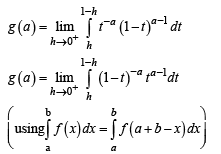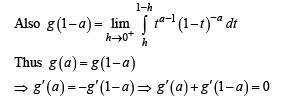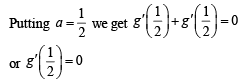*Multiple options can be correct
Test: Comprehension Based Questions: Definite Integrals and Applications of Integrals - Question 15

PASSAGE - 6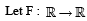be a thrice differentiable function. Suppose that F(1) = 0, F(3) = –4 and F(x) < 0 for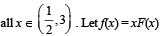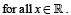Q. The correct statement(s) is(are)

Detailed Solution for Test: Comprehension Based Questions: Definite Integrals and Applications of Integrals - Question 15

f(x) = xF(x) ⇒ f ' (x) = F(x) + xF '(x)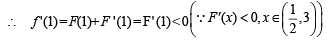f(2) = 2F(2) < 0,

(Q∵F '(x) < 0 ⇒ F is decreasing on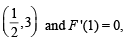F(3) = –4)
f '(x) = F(x) + x F '(x)

For the same reason given above and F '(x) < 0 given.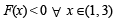∴ f '(x) ≠ 0, x∈(1, 3)

*Multiple options can be correct
Test: Comprehension Based Questions: Definite Integrals and Applications of Integrals - Question 16

PASSAGE - 6be a thrice differentiable function. Suppose that F(1) = 0, F(3) = –4 and F(x) < 0 forQ.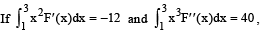Detailed Solution for Test: Comprehension Based Questions: Definite Integrals and Applications of Integrals - Question 16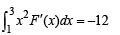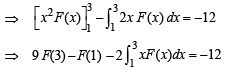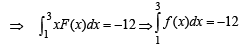...(i)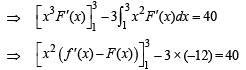⇒ 9(f ' (3) – F(3)) – (f ' (1) – F(1)) = 4
⇒ 9f ' (3) – 9 × (–4) – f ' (1) + 0 = 4
⇒ 9f ' (3) – f ' (1) + 32 = 0

## Maths 35 Years JEE Main & Advanced Past year Papers

132 docs|70 tests
 Use Code STAYHOME200 and get INR 200 additional OFF Use Coupon Code
Information about Test: Comprehension Based Questions: Definite Integrals and Applications of Integrals Page
In this test you can find the Exam questions for Test: Comprehension Based Questions: Definite Integrals and Applications of Integrals solved & explained in the simplest way possible. Besides giving Questions and answers for Test: Comprehension Based Questions: Definite Integrals and Applications of Integrals, EduRev gives you an ample number of Online tests for practice

## Maths 35 Years JEE Main & Advanced Past year Papers

132 docs|70 tests